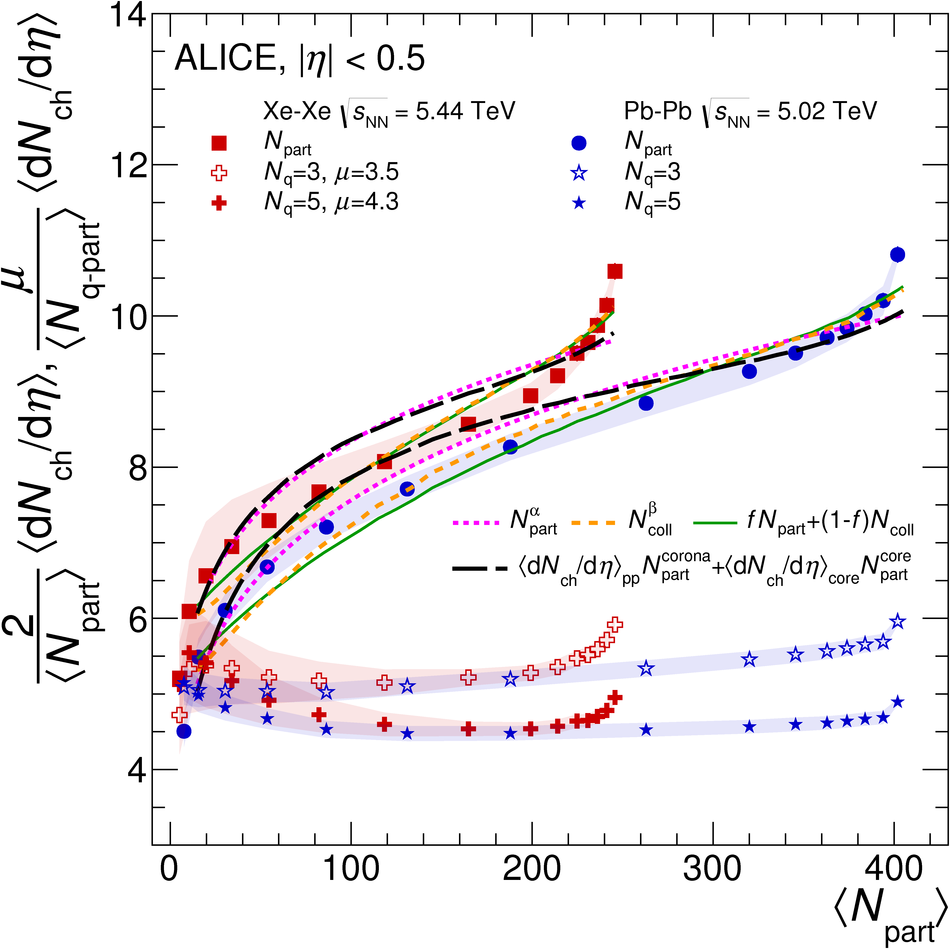# Figure 5

 The $\dNdetape$ for \XeXe\ collisions at \snn~=~\unit[5.44]{TeV} and \PbPb\ collisions at \snn~=~\unit[5.02]{TeV}~, as a function of $\langle N_{\mathrm{part}} \rangle$. The \PbPb\ data are fitted with various parameterisations of \Npart\ and \Ncoll, calculated with the Glauber model The same functions, with the values of the parameters from the Pb--Pb fit, are used for the \XeXe\ data Also shown is \dNdeta\ per participant quark, \Ncpart, calculated with the effective wounded constituent quarks model~, as a function of \avNpart. The number of participant quarks \Ncpart\ is normalised by the average number of participant quarks in \pp\ collisions, $\mu$.